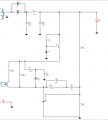# How Power Supply Limit its Current Output ?

Joined Sep 28, 2021
40In the picture above, how does the power supply limit its max current and it has been fascinating for me to figure out how. Does anyone have a clue on this? I just know that the capacitor and diode help to rectify the AC output of transformer to DC current, but how does the other parts help to limit its max output current?

Thank you and have a nice day#### Ian0

Joined Aug 7, 2020
3,754
It's in the part of the drawing you have missed off.
The current in the MOSFET is limited. That limits the current in the inductor. The energy stored in the inductor is
$$E=\frac {1}{2} I_{MOSFET}^2L$$
If the energy supplied per cycle is limited then the power is limited to
$$P=\frac {1}{2} I_{MOSFET}^2Lf$$

$$E=\frac {1}{2} I_{MOSFET}^2L$$
$$P=\frac {1}{2} I_{MOSFET}^2Lf$$